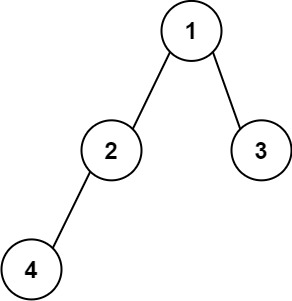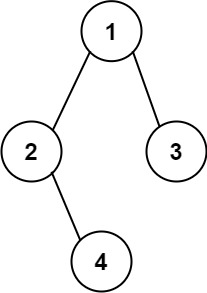### LeetCode• ㊗️
• 大家
• offer
• 多多！

## Problem

Given the root of a binary tree, construct a string consisting of parenthesis and integers from a binary tree with the preorder traversal way, and return it.

Omit all the empty parenthesis pairs that do not affect the one-to-one mapping relationship between the string and the original binary tree.

Example 1:Input: root = [1,2,3,4]
Output: "1(2(4))(3)"
Explanation: Originally, it needs to be "1(2(4)())(3()())", but you need to omit all the unnecessary empty parenthesis pairs. And it will be "1(2(4))(3)"


Example 2:Input: root = [1,2,3,null,4]
Output: "1(2()(4))(3)"
Explanation: Almost the same as the first example, except we cannot omit the first parenthesis pair to break the one-to-one mapping relationship between the input and the output.


Constraints:

• The number of nodes in the tree is in the range $[1, 10^4]$.
• -1000 <= Node.val <= 1000

## Code

class Solution {
public String tree2str(TreeNode root) {
if (root == null) return "";

String res = root.val + "";

String left = tree2str(root.left);
String right = tree2str(root.right);

if (left == "" && right == "") return res;

if (left == "") return res + "()" + "(" + right + ")";
if (right == "") return res + "(" + left + ")";

return res + "(" + left + ")" + "(" + right + ")";
}
}